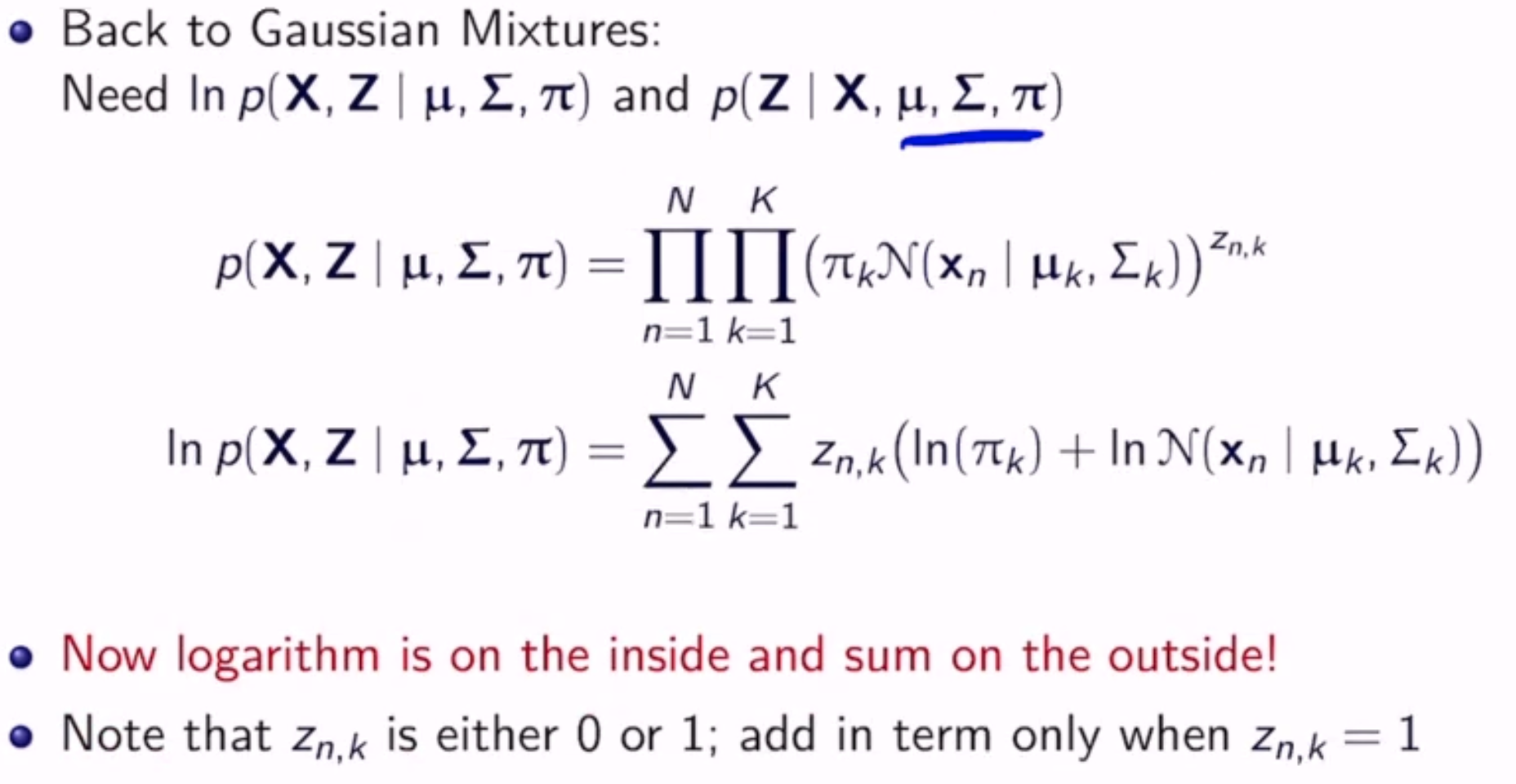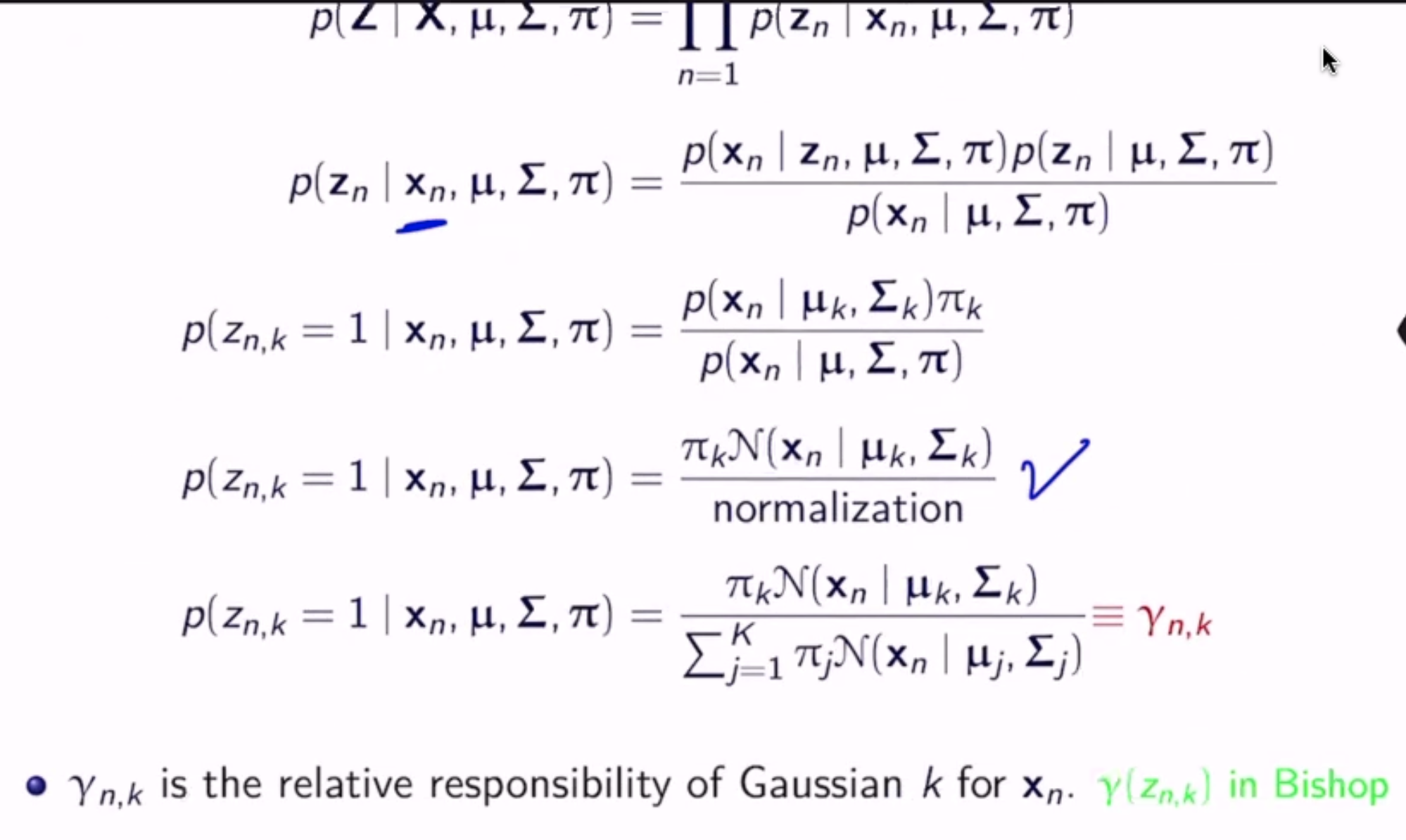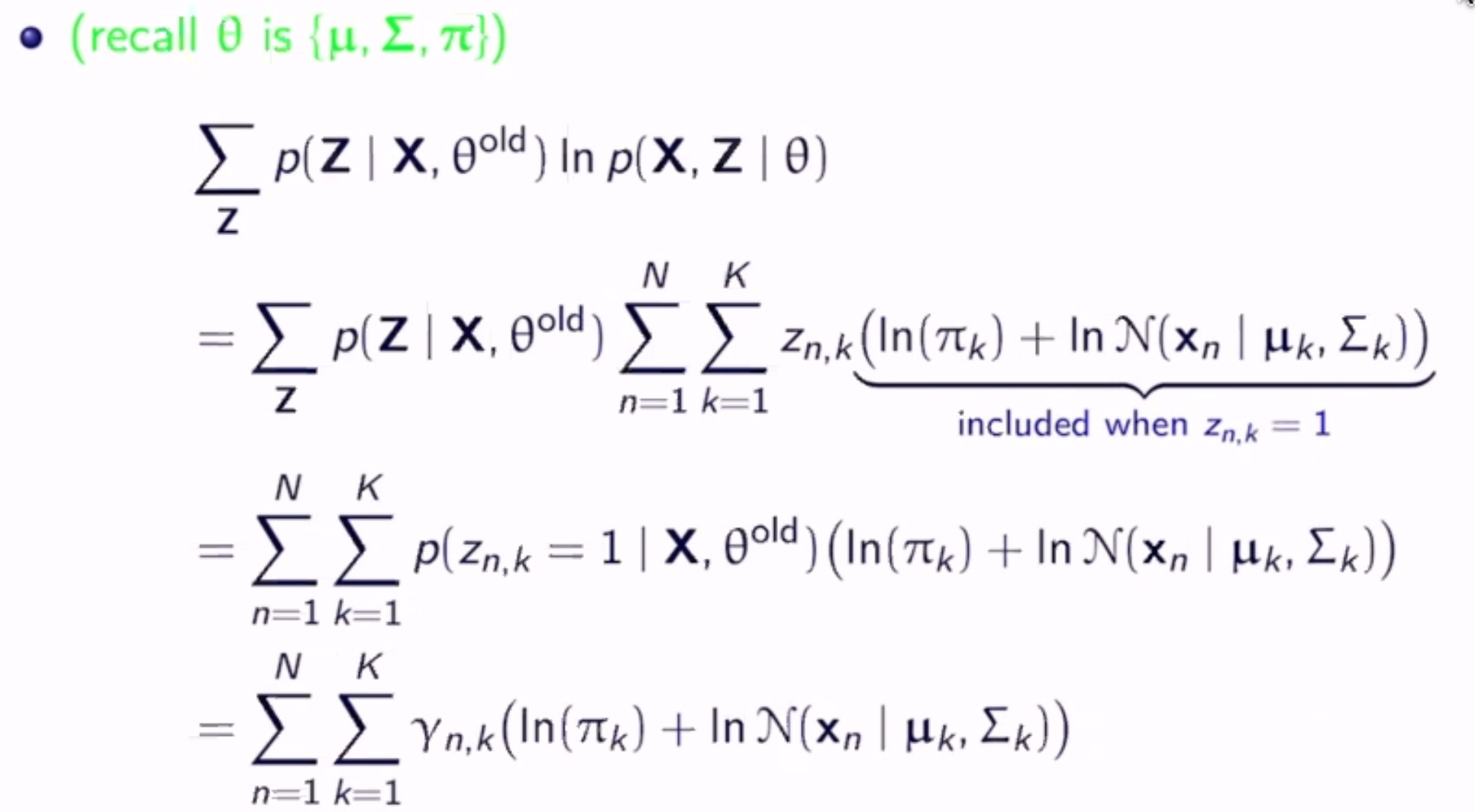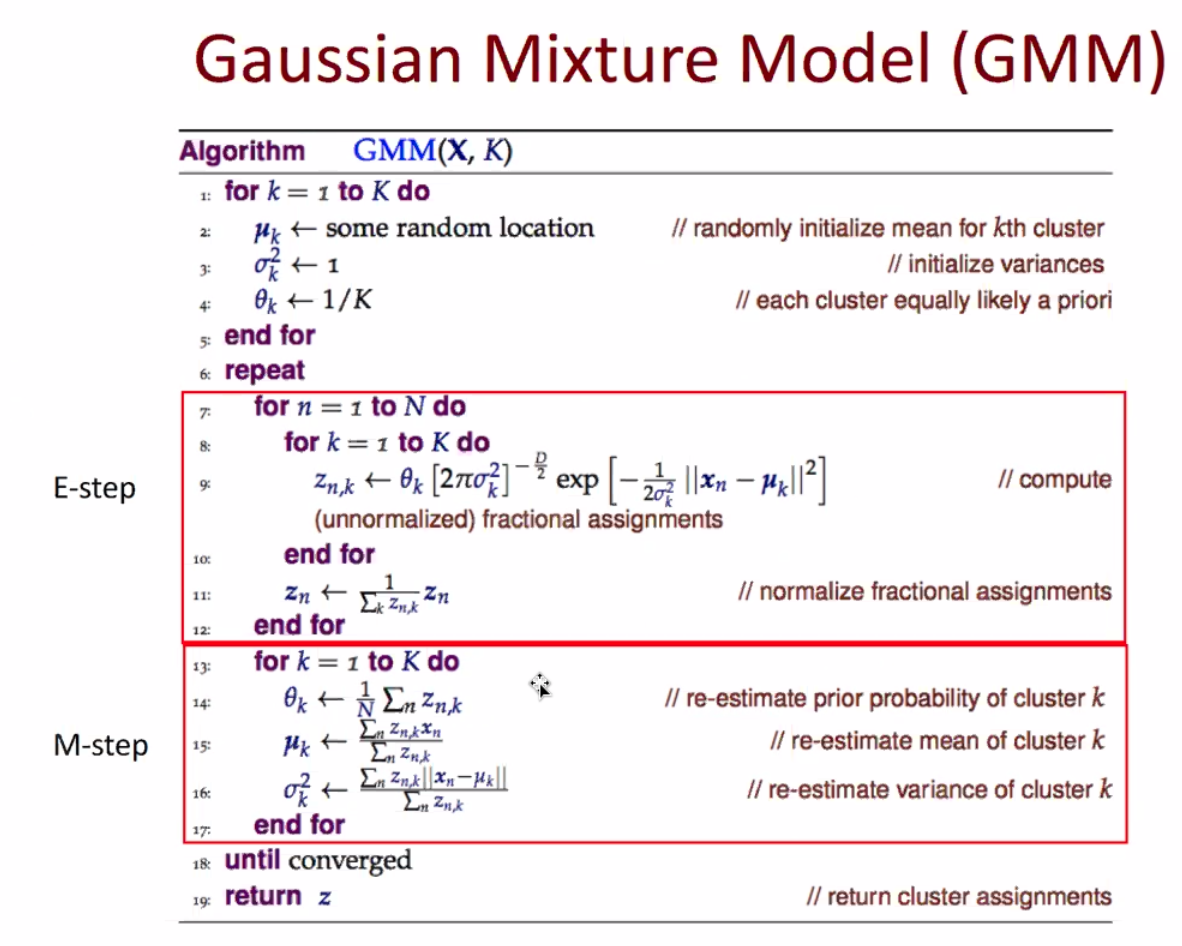# EM/GMM¶

Gaussian Mixture Models: Estimate Mixtures of $$K$$ Gaussians

• pick sample from gaussian $$k$$ with prob. $$\pi_k$$
• generative distribution $$p(\mathbf{x}) = \sum_{k=1}^K \pi_k N(\mathbf{x} | \mathbf{\mu}_k, \mathbf{\Sigma}_k)$$
• where $$N$$ is the gaussian distibution
• a mixture distribution with mixture coefficients $$\mathbf{\pi}$$
• for iid sample $$\mathbf{X}$$ and parameters $$\theta = \{\mathbf{\pi}, \mathbf{\mu}, \mathbf{\Sigma}\}$$, we have:
$\begin{split}p(\mathbf{X} | \mathbf{\pi}, \mathbf{\mu}, \mathbf{\Sigma}) & = \prod_{n=1}^N p(\mathbf{x_n} | \mathbf{\pi}, \mathbf{\mu}, \mathbf{\Sigma}) \\ & = \prod_{n=1}^N \sum_{k=1}^K \pi_k N(\mathbf{x} | \mathbf{\mu}_k, \mathbf{\Sigma}_k)\end{split}$

What is $$\theta$$?

## Log-Likelihood¶

$\begin{split}L(\pi, \mu, \Sigma) & = \ln p(\mathbf{X} | \mathbf{\pi}, \mathbf{\mu}, \mathbf{\Sigma}) \\ & = \ln (\prod_{n=1}^N \sum_{k=1}^K \pi_k N(\mathbf{x} | \mathbf{\mu}_k, \mathbf{\Sigma}_k)) \\ & = \sum_{n=1}^N \ln (\sum_{k=1}^K \pi_k N(\mathbf{x} | \mathbf{\mu}_k, \mathbf{\Sigma}_k))\end{split}$

This is very hard to solve directly!

## Iteratively¶

• which gaussian picked for $$x_i \in X$$ is a latent variable $$z_i$$ in $$\{0, 1\}^K$$ (one of K encoding)
• $$Z$$ is vector of $$z_i$$’s
• note that $$p(\mathbf{X} | \mathbf{\pi}, \mathbf{\mu}, \mathbf{\Sigma}) = \sum_Z p(\mathbf{X}, Z | \mathbf{\pi}, \mathbf{\mu}, \mathbf{\Sigma})$$
• complete data is $$\{X, Z\}$$
• incomplete data is just $$X$$
• don’t know $$Z$$, but from $$\theta^{old}$$ can inferMaximize this to get the new parameters.